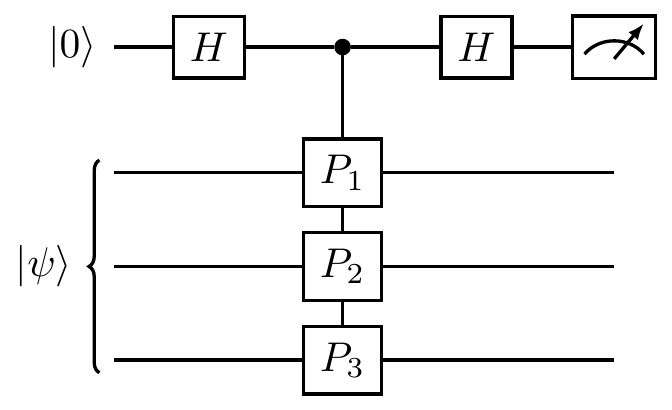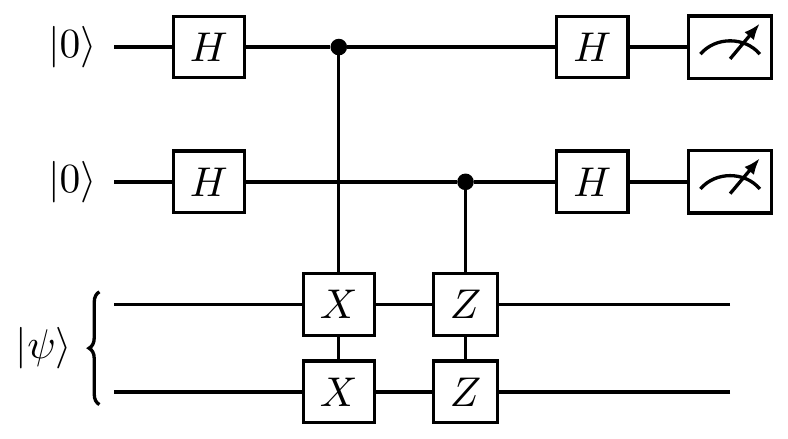## 10.4 Oracles, and our first quantum algorithm

The computational power of quantum interference was discovered by counting how many times certain Boolean functions have to be evaluated in order to find the answer to a given problem. Imagine a “black box” (sometimes also called an oracle) that computes some fixed Boolean function, but whose inner workings are unknown to us. Then imagine that we are in a scenario where we want to learn about some given property of the Boolean function, but we have to “pay” (in energy, time, money, or anything!) for each use (often referred to as a query) of the box. In such a setting, the objective is to minimise number of queries to the oracle while finding out as much information as possible about the function that it computes. For this purpose, we ignore everything that happens inside the black box: in our rules of the game, the Boolean function evaluation counts as just one computational step.

### 10.4.1 Deutsch’s algorithm

We start, once more, with the simplest quantum interference circuitbut let’s turn this into a black-box scenario, as described above:

• we are allowed to prepare the input in any state that we like;
• we are allowed to read the output;
• but all we know about the value of \varphi is that it is either 0 or \pi, and we are not allowed to “look inside” the phase gate to see which value it is!

With these rules, can we figure out which value \varphi has been set to?

Of course we can — we’re quantum information scientists!

One way of doing it is to prepare the input in the state |0\rangle and check the output: if \varphi=0 then the output is always |0\rangle, and if \varphi=\pi then it is always |1\rangle. In other words, a single run of the interference experiment is sufficient to determine the difference.

The very first quantum algorithm, proposed by David Deutsch in 1985, is very much related to this effect, but where the phase setting is determined by the Boolean function evaluation via the phase kick-back.

We are presented with an oracle that computes some unknown function f\colon\{0,1\}\to\{0,1\}. Note that there are only four possibilities for what f can be: it could be one of two constant functions (i.e. those where f(0)=f(1)), or one of two balanced functions (i.e. those where f(0)\neq f(1)).

f(0) f(1)
constant 0 0
constant 1 1
balanced 0 1
balanced 1 0

Our task is to determine, using the fewest queries possible, whether the function computed by the oracle is constant or balanced.

Note that we are not asked for the particular values f(0) and f(1), but only whether the two values are the same or different. Classical intuition tells us that we have to evaluate both f(0) and f(1) and compare them, which involves evaluating f twice. But, in the quantum setting, we can solve this problem with a single function evaluation, using the following circuit.166

(Deutsch’s).

First register: 1 qubit. Second register: 1 qubit.During the function evaluation, the second register “kicks back” the phase factor (-1)^{f(x)} in front of |x\rangle, but the state of the second register remains unchanged; the first register is modified as follows: \begin{aligned} |0\rangle &\overset{H}{\longmapsto} |0\rangle+|1\rangle \\&\overset{U_f}{\longmapsto} (-1)^{f(0)}|0\rangle + (-1)^{f(1)}|1\rangle \\&\quad= |0\rangle + (-1)^{f(0)\oplus f(1)}|1\rangle \\&\overset{H}{\longmapsto} |f(0)\oplus f(1)\rangle. \end{aligned}

The evolution of the first qubit is thus identical to that described by the circuit diagramwhere the relative phase is \varphi = (-1)^{f(0)\oplus f(1)}. The first qubit ends in state |0\rangle if the function f is constant, and in state |1\rangle if the function is balanced, and the standard measurement distinguishes these two cases with certainty.167

Deutsch’s result laid the foundation for the new field of quantum computation, and was followed by several other quantum algorithms for various problems. They all seem to rest on the same generic sequence:

• a Hadamard transform;
• function evaluation;
• another Hadamard (or Fourier) transform.168

As we shall see in a moment, in some cases (such as in Grover’s search algorithm) this sequence is repeated several times.

Let us now follow a tour through the three early quantum algorithms, where each one offers a higher-order speed-up when compared to their classical analogues than the last: firstly linear, then quadratic, and finally exponential.

1. The original Deutsch algorithm provides the correct answer with probability 50%. Here we present a modified/improved version. The more general problem, which deals with unknown functions f\colon\{0,1\}^n\to\{0,1\} for n\geqslant 1, is known as the Deutsch–Jozsa problem.↩︎

2. This is also implemented in the Quantum Flytrap Virtual Lab.↩︎

3. As explained in Section 10.2, the Hadamard transform is a special case of the Fourier transform over the group \mathbb{Z}_2^n.↩︎